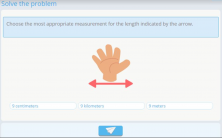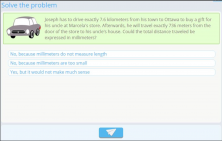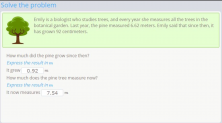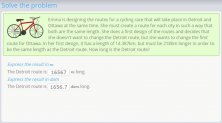Smartick is an advanced online program that teaches kids math and coding in only 15 min. a day
Try it for free!

Aug11

# Practice Length Measurement Problems

To solve length measurement problems, we need to have various factors in mind: the unit of measurement used, conversion exercises, and the understanding of how to use different measuring units. In this post, we’ll present different exercise types that’ll help us understand these concepts.

### Unit of Measure Used to Express the Information

In problems that deal with length measurements, it’s extremely important to always have the unit that is used in mind. A length expressed in km is not the same thing as a length expressed in cm.

To stress the importance of this concept, it’s important to do exercises that make us approximate the measuring unit according to the length of the object that is being measured.This is an example of a problem where we work on the approximation of measurements according to the unit in use.

These measurements don’t have to be exact because the exact measurement is not what we’re after. What’s important is that the student understands that, in this case, a palm can’t be measured in kilometers or meters, but rather, in the appropriate unit, or centimeters.

### Understanding the Use of Different Measuring Units

It’s also important to show students the practicality of this lesson with problems where they have to apply what they’ve learned.This is how we can teach the practicality of expressing each situation according to its corresponding unit of measure. It also helps you be more attentive to the differences so you can go ahead with changing the units necessary in order to solve the problem.

### Conversion Length Measurement Problems

The problems that we’ve made for the application on unit conversion have to be realistic so that they teach students how to apply what they’ve learned in real life.So, wrapping up, we maintain that in order to do exercises that require students to know length units, we must continue the learning process with different concepts that are implied by this topic in a progressive manner:

1. The practicality of using different length and measuring unit multiples and submultiples.
2. Making examples out of different real-life situations.
3. Applying what was learned to real-life situations that require unit conversions. If you thought this post was interesting, remember that you can find more like this at Smartick

Learn More: